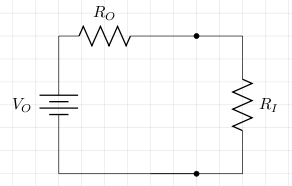# Maximum Power Transfer

The circuit below is a Thevenin equivalent of an output device (VO, RO) and an input device. Let the input resistor RI be the load for the output.The power dissipated in RI, PI=I2RI. The power dissipated in RO, PO=I2RO. The power generated by VO=IVO. The current is I=VO/(RI+RO). So,

```PI=I2RI.
=(VO2/(RI+RO)2)RI.
```

The maximum (dPI/dRI=0) is at RI=RO and this is the maximum power.

• Maximum power RI: RI=RO
• Maximum voltage drop across RI: RI>>RO
• Maximum current RI: RI<<RO

## References

Fischer-Cripps. A.C., The Electronics Companion. Institute of Physics, 2005.